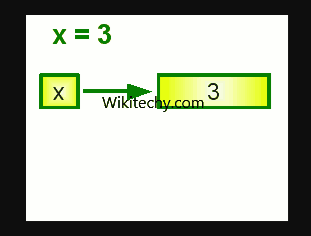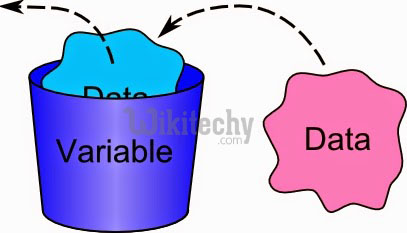# C# Global Variable | C# Variable - c# - c# tutorial - c# net

## What is variable in C# ?

• A variable is a name of memory location. It is used to store data. Its value can be changed and it can be reused many times.
• It is a way to represent memory location through symbol so that it can be easily identified.
• The basic variable type available in C# can be categorized as:

• Variable Type Example
Decimal types decimal
Boolean types True or false value, as assigned
Integral types int, char, byte, short, long
Floating point types float and double
Nullable types Nullable data types## Syntax to declare a variable:

• The example of declaring variable is given below:
• int i, j;
• double d;
• float f;
• char ch;
• Here, i, j, d, f, ch are variables and int, double, float, char are data types.
• We can also provide values while declaring the variables as given below:
• int i=2,j=4; //declaring 2 variable of integer type
• float f=40.2;
• char ch='B';

## Rules for defining variables

• A variable can have alphabets, digits and underscore.
• No white space is allowed within variable name.
• A variable name must not be any reserved word or keyword e.g. char, float etc.• int x;
• int _x;
• int k20;

## Invalid variable names:

• int 4;
• int x y;
• int double;learn c# tutorial - class-object-contructor-member-function-in-csharp in c# Example